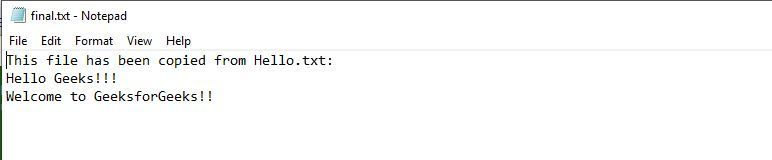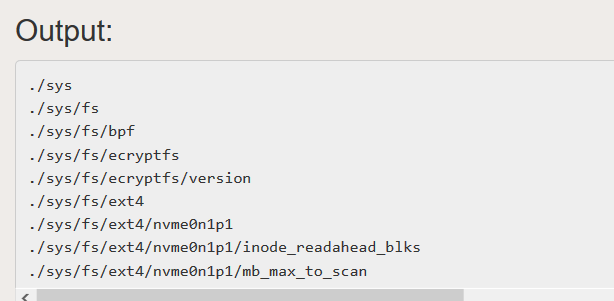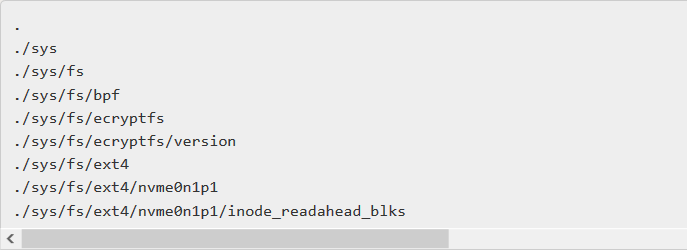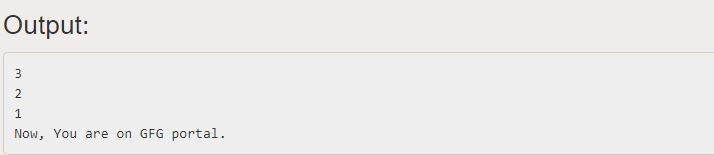GeeksforGeeks App
Open AppBrowser
Continue

# Perl | Recursive Subroutines

Prerequisite: Recursion in Perl
Recursive means pertaining to or using a rule or procedure that can be applied repeatedly. It is the process of defining a function or calculating a number by the repeated application of an algorithm. Recursive Subroutine is a type of subroutine that calls itself as part of its execution or is in a potential cycle of function calls. Perl provides us with the flexibility to use subroutines both iteratively and recursively.
A simple example showing the use of recursive subroutine in Perl would be that calculating the factorial of a number.
Example :

## Perl

 `#!/usr/bin/perl``  ` `# Perl Program to calculate Factorial ``sub` `factorial``{``      ` `my` `\$n` `= ``\$_``;``  ` `# checking if that value is 0 or 1``if` `(``\$n` `== 0 || ``\$n` `== 1)``{``    ``return` `1;``}``  ` `# Recursively calling the function with the next value``# which is one less than current one``else``{``    ``return` `\$n` `* factorial(``\$n` `- 1);``}``}``  ` `# Driver Code``\$n` `= 7;``  ` `# Function call and printing result after return``print` `"Factorial of a number ``\$n` `is ", factorial(``\$n``);`

Output:

`Factorial of a number 7 is 5040`

### Traversing a Directory Tree

Traversing a directory tree means to iterate over or to print each file and sub-directories within a root directory. The directory tree is a representation of the sub-directories and files within sub-directories and other files within the directory in the form of a tree denoting the parent-child relationship between these directories and respective files.
Both Unix and Windows systems organize file directories into a tree structure. Although in Perl, traversing a directory tree or walking a directory tree can be done both iteratively and recursively, but we often use the later one when the number of files or sub-directories inside our root folder is very large. This is because of the fast and less number of lines of code written in recursive function in comparison to an iterative one. The later is used when the corresponding number of files are less in number.
We’ll use File::Find to traverse the file system iteratively and collect the filenames.

Syntax :
use File::Find;
find(\&wanted, @directories_to_search);
sub wanted { … }
use File::Find;
finddepth(\&wanted, @directories_to_search);
sub wanted { … }
use File::Find;
find({ wanted => \&process, follow => 1 }, ‘.’);

Example :

## Perl

 `#!/usr/bin/perl``use` `strict;``use` `warnings;``    ` `use` `File::Find::Rule;``use` `File::Basename ``qw(basename)``;``    ` `my` `\$loc` `= "C:\Users\GeeksForGeeks";``my` `\$file` `= ``'final.txt'``;``my` `\$expected` `= ;``chomp` `\$expected``;``    ` `open``(``my` `\$fh``, ``'<'``, ``\$expected``) or``die` `"Could not ``open` `'\$expected'` `\$!\n";` `open``(``my` `\$out``, ``'>'``, ``\$file``) or``die` `"Could not ``open` `'\$file'` `\$!\n";``    ` `my` `@paths` `= File::Find::Rule->file->name(``'*.pdf'``)->in(``\$loc``);``my` `@files` `= ``map` `{ ``lc` `basename ``\$_` `} ``@paths``;``my` `%case` `= ``map` `{ ``\$_` `=> 1 } ``@files``;``    ` `print` `\$out` `"This file ``has` `been copied from (``\$loc``)``\$file``:\n";``while` `(``my` `\$name` `= <``\$fh``>)``{``    ``chomp` `\$name``;``    ``if` `(``\$case``{``lc` `\$name``})``    ``{``        ``print` `"``\$name` `found\n";``    ``}``    ``else``    ``{``        ``print` `\$out` `"``\$name``\n";``    ``}``}``close` `\$out``;``close` `\$fh``;`

Output: Source file gets copied to the destination fileIn the coming section, we will discuss the Recursive traversal of the root directory.

### Recursively Traversing a Directory Tree

The problem with the iterative solution of traversal apart from its slow speed and more lines of code is the fact that all the sub-directories within the root directory must have the same orientation and the same number of files within them. Otherwise, the traversal can become a complex task. Recursive solution plays a better role in overcoming the problem with the iterative solution.
Example (Recursive) :

## Perl

 `#!/usr/bin/perl` `# Perl recursive Program to walk``# through a directory tree``use` `strict;``use` `warnings;``use` `5.010;` `my` `\$loc` `= ``shift` `|| ``'.'``;` `# Calling walk subroutine with``# the location of the root directory``walk(``\$loc``);` `# Subroutine definition``sub` `walk``{``    ``my` `(``\$case``) = ``@_``;` `    ``# If case is not a directory itself``    ``return` `if` `not -d ``\$case``;``    ` `    ``# If case is a directory``    ``opendir` `my` `\$dh``, ``\$case` `or ``die``;` `    ``while` `(``my` `\$sub` `= ``readdir` `\$dh``)``    ``{``        ``next` `if` `\$sub` `eq ``'.'` `or ``\$sub` `eq ``'..'``;``        ``say` `"``\$case``/``\$sub``";``        ``walk("``\$case``/``\$sub``");``    ``}``    ``close` `\$dh``;``    ``return``;``}`

Output :The above code will let you loop over each file in the root directory and all the sub-directories present in the root directory along with the files and directories within these sub-directories.

### Top Down Approach

The top-down approach basically divides a complex problem or algorithm into multiple smaller parts (modules). These modules are further divided until the resulting module is a program which can not be further decomposed.
Here, we first initialize a variable called \$case which keeps hold of the file or the directory being iterated or looped over. Then if it’s a file, then the function simply prints its name and loop over to next file or directory or else if the \$case is a directory, then it calls the subroutine again with the directory location as the current directory location and then loop over all the files and sub-directories within that directory. After the completion of the inner function, the pointer returns back to the location of the outer directory and print its name and then moves to the next file or the directory present in the root directory. This is actually a typical Top-Down Approach used in Perl for traversing or walking over a directory tree.
Example :

## Perl

 `use` `strict;``use` `warnings;``use` `5.010;` `my` `\$loc` `= ``shift` `|| ``'.'``;` `walk(``\$loc``);` `sub` `walk``{``    ``my` `(``\$case``) = ``@_``;``    ` `    ``say` `\$case``;``    ``return` `if` `not -d ``\$case``;``    ``opendir` `my` `\$dh``, ``\$case` `or ``die``;``    ``while` `(``my` `\$sub` `= ``readdir` `\$dh``)``    ``{``        ``next` `if` `\$sub` `eq ``'.'` `or ``\$sub` `eq ``'..'``;` `        ``walk("``\$case``/``\$sub``");``    ``}``    ``close` `\$dh``;``    ``return``;``}`

Output :The code explained in the section Recursively traversing a directory tree is an example of the Top-Down Approach used in Perl.

Note : The above Output image shows a small portion of the complete output as there are a lot of files and subdirectories inside the root directory. This code works fine for folders and directories with a small number of sub-directories.

### Recursive Function

A recursive function has the following general form:

Function( arguments ) {
if a simple case, return the simple value // base case / stopping condition
else call function with simpler version of problem

Example :

## Perl

 `#!/usr/bin/perl` `# Perl Program to calculate Factorial``sub` `myfunc``{``    ``my` `\$n` `= ``\$_``;``    ` `    ``# checking if that value is``    ``# greater than 0 or not``    ``if` `(``\$n` `<= 0)``    ``{``        ``print` `"Now, You are on GFG portal.\n";``    ``}``    ` `    ``# Recursively calling function with``    ``# the next value which is one less``    ``# than current one``    ``else``    ``{``        ``print` `"``\$n``\n";``        ``myfunc(``\$n` `- 1);``    ``}``}` `# Driver Code` `# Function call``myfunc(3);`

Output:For a recursive function to stop calling itself we require some type of stopping condition. If it is not the base case, then we simplify our computation using the general formula. A recursive function has two major parts. The first one checks for some condition and returns if that condition was met. This is called the halting condition, or stop condition. Then at some later point in the function, it calls itself with a different set of parameters than it was called earlier.

#### Why recursion preferred over iteration ?

1. It comes with the positives of writing cleaner and simpler short code.
2. It is also efficient in terms of time if used with memorization
3. Performs better in solving problems based on tree structures.

My Personal Notes arrow_drop_up